Processing ......FreeComputerBooks.com Free Computer, Mathematics, Technical Books and Lecture Notes, etc.

Financial Mathematics, Financial Engineering, and Financial Technologies
Related Book Categories:
 Statistics and SAS Data Analysis and Data Mining Mathematical Software, MATLAB Numerical Analysis and Computing Calculus and Mathematical Analysis Machine LearningProbability and Stochastic Processes The R Programming Combinatorics and Game Theory Applied Mathematics Algebra and Abstract Algebra Finance Research Library•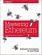Mastering Ethereum: Building Smart Contracts and DApps

The book is intended to serve both as a reference manual and as a cover-to-cover exploration of Ethereum. If you're looking to get started with the Ethereum protocol (open source developers, integrators, etc.), this is the definitive book on the topic.

•Mastering Bitcoin: Programming the Open Blockchain

This book is your guide through the seemingly complex world of Bitcoin, providing the knowledge you need to participate in the internet of money. It will help you engineer money. You're about to unlock the API to a new economy. This book is your key.

•Mathematical Methods for Economic Theory (Martin J. Osborne)

This book covers the basic mathematical tools used in economic theory. It emphasizes techniques rather than abstract theory. However, the conditions under which each technique is applicable are stated precisely.

•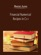Financial Numerical Recipes in C++: Applications in Finance

This book provides a good deal of useful examples and algorithms for people working within the field of finance, in C++. All the routines have been made to confirm to the new ISO/ANSI C++ standard, using namespaces and the standard template library.

•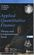Applied Quantitative Finance: Theory and Computational Tools

This book presents solutions, theoretical developments and method proliferation for many practical problems in quantitative finance. The combination of practice and theory supported by computational tools is reflected in the selection of topics.

•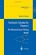Stochastic Calculus and Finance (Steven E. Shreve)

The book gives both precise statements of results, plausibility arguments, and even some proofs, but more importantly intuitive explanations developed and refine through classroom experience with this material are provided.

•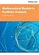Mathematical Models in Portfolio Analysis (Farida Kachapova)

This book explains portfolio modelling in financial mathematics as a consistent mathematical theory with all steps justified. The topics include mean-variance portfolio analysis and capital market theory. The book contains many examples with solutions.

•Numerical Methods for Finance (Robert Nurnberg)

This book explores new and relevant numerical methods for the solution of practical problems in finance. It is one of the few books entirely devoted to numerical methods as applied to the financial field.

•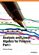Analysis and Linear Algebra for Finance (Patrick Roger)

This book presents the elements of analysis and linear algebra used in financial models and in microeconomics. Functions of one and several variables and matrices are developed as well as vector spaces, linear mappings and optimization methods, etc.

•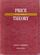Price Theory: An Intermediate Text (David D. Friedman)

This book was designed to develop the reader's understanding of the economic way of thinking by first providing verbal, intuitive explanations of concepts, then illustrating them with graphs and/or calculus..

•Games, Fixed Points and Mathematical Economics (C. Ewald)

This book gives the reader access to the mathematical techniques involved and goes on to apply fixed point theorems to proving the existence of equilibria for economics and for co-operative and noncooperative games.

•Portfolio Theory and Financial Analyses (Robert Alan Hill)

This book connects each of the major categories of techniques and practices to the unifying and seminal conceptual developments of modern portfolio theory, whether these involve measuring the return on a portfolio, analysing portfolio risk or evaluating the quality of the portfolio management process.

•Financial Applications using Excel Add-in in C/C++ (Steve Dalton)

This book is a must-buy book for any serious Excel developer, the only complete how-to guide and reference book for the creation of high performance add-ins for Excel in C and C++ for users in the finance industry.

•Stochastic Processes for Finance (Patrick Roger)

It describes the most important stochastic processes used in finance in a pedagogical way, especially Markov chains, Brownian motion and martingales. It also shows how mathematical tools like filtrations, Ito's lemma or Girsanov theorem should be understood in the framework of financial models.

•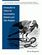Python for Econometrics, Statistics and Data Analysis

This book provides an introduction to Python for a beginning programmer. They may also be useful for an experienced Python programmer interested in using NumPy, SciPy, and matplotlib for numerical and statistical analaysis.

•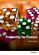Probability for Finance (Patrick Roger)

This book provides technical support for students in finance. It reviews the main probabilistic tools used in financial models in a pedagogical way, starting from simple concepts like random variables and tribes and going to more sophisticated ones like conditional expectations and limit theorems.

•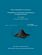From Algorithms to Z-Scores: Probabilistic and Statistical Modeling

This is a textbook for a course in mathematical probability and statistics for computer science students.

•Basic Data Analysis and More - A Guided Tour Using Python

In this book, a selection of frequently required statistical tools will be introduced and illustrated.

•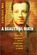A Beautiful Math: John Nash, Game Theory, and a Code of Nature

At the time of Nash's early work, game theory was briefly popular among some mathematicians and Cold War analysts.

•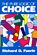The Pure Logic Of Choice (Richard D. Fuerle)

This book presents a general theory of economics based on free will. The laws of economics are deduced from the premise that people have free will and can change physical things in an attempt to achieve their chosen values.

•Accounting Succinctly: A Developer's Guide (Joe Booth)

This book is a developer's guide to basic accounting. Written with business app development in mind, it discusses some of the most common accounting processes, including assets, multiple accounts, journaling, posting, inventory, and payroll.

•DevOps for Finance (Jim Bird)

Aims to debunk that myth by showing how the finance industry can benefit from DevOps practices and perform a greater degree of automation by implementing DevOps properly. It explains DevOps benefits that are far more important for the financial services industry.

•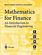Mathematics for Finance: An Introduction to Financial Engineering

This book is an excellent introduction to Mathematical Finance. Armed with a knowledge of basic calculus and probability a student can use this book to learn about derivatives, interest rates and their term structure and portfolio management.

•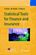Statistical Tools for Finance and Insurance (Pavel Cizek, et al)

This book presents ready-to-use solutions, theoretical developments and method construction for many practical problems in quantitative finance and insurance.

•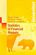Statistics of Financial Markets: An Introduction (Jürgen Franke)

The focus is both on fundamentals of mathematical finance and financial time series analysis and on applications to given problems of financial markets.

•Strategic Foundations of General Equilibrium: Bargaining Games

Making use of insights from game theory, search theory and bargaining theory, the author develops a model to explain what actually goes on in markets and how a competitive general equilibrium is achieved.

•Financial Mathematics and Financial Engineering

This is the previous page of Financial Mathematics and Financial Engineering, we are in the processing to convert all the books there to the new page. Please check this page daily!!!

Book CategoriesAll CategoriesRecent BooksIT Research LibraryMiscellaneous Books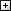Computer LanguagesComputer ScienceData Science/DatabasesElectronic EngineeringJava and Java EE (J2EE)Linux and UnixMathematicsMicrosoft and .NETMobile ComputingNetworking and CommunicationsSoftware EngineeringSpecial TopicsWeb Programming
Other Categories